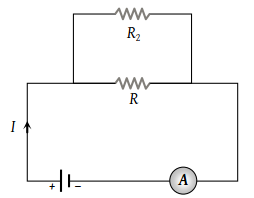• Subject:

...

• Topic:

...

If a resistance R2 is connected in parallel with the resistance R in the circuit shown, then possible value of current through R and the possible value of R2 will be(1) $\frac{I}{3},\text{\hspace{0.17em}}R$

(2) $I,\text{\hspace{0.17em}}2R$

(3) $\frac{I}{3},\text{\hspace{0.17em}}2R$

(4) $\frac{I}{2},\text{\hspace{0.17em}}R$